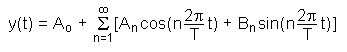The SDL Component Suite is an industry leading collection of components supporting scientific and engineering computing. Please visit the SDL Web site for more information....## FourSerSinCoeff

 Unit: SDL_fourier Class: TFastFourier Declaration: property FourSerSinCoeff[n: integer]: double;

The readonly array property FourSerSinCoeff returns the coefficients Bn of the sine terms of the Fourier series (cf. to equation below). The index n may assume values between 1 and SpectrumSize div 2. Accessing any value of the array below 1 and above SpectrumSize will result in a zero value on read, or in no action on write.By using the above equation and the properties FourSerSinCoeff and FourSerCosCoeff the original curve can be reconstructed after a Fourier transform has been performed (of course, an inverse transform would do the same).

 Hint 1: In order to obtain "valid" results the property WeightingWindow has to be set to fwRectangle.

 Hint 2: Mind the subtle difference between the Magnitude and the Fourier series coefficients. The Magnitude does not preserve the signs, while the properties FourSerSinCoeff and FourSerCosCoeff do.

 Hint 3: FourSerSinCoeff could be easily calculated from ImagSpec by subtracting the coefficient of the corresponding negative frequency from the coefficient of the positive frequency.

 Hint 4: The index n into the property starts from zero (as opposed to the other array properties)

 Example: This property is used in the following example program (see http://www.lohninger.com/examples.html for downloading the code): fft

Last Update: 2012-Oct-20